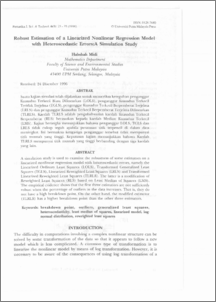# Robust Estimation of a Linearized Nonlinear Regression Model with Heteroscedastic Errors:A Simulation Study

## Citation

Midi, Habshah (1998) Robust Estimation of a Linearized Nonlinear Regression Model with Heteroscedastic Errors:A Simulation Study. Pertanika Journal of Science & Technology, 6 (1). pp. 23-35. ISSN 0128-7680

## Abstract

A simulation study is used to examine the robustness of some estimators on a linearized nonlinear regression model with heteroscedastic errors, namely the Linearized Ordinary Least Squares (LOLS), Transformed Generalized Least Squares (TGLS) , Linearized Reweighted Least Squares (LRLS) and Transformed Linearized Reweighted Least Squares (TLRLS). The latter is a modification of Reweighted Least Squares (RLS) based on Least Median of Squares (LMS). The empirical evidence shows that the first three estimators are not sufficiently robust when the percentage of outliers in the data increases. That is, they do not have a high breakdown point. On the other hand, the modified estimator (TLRLS) has a higher breakdown point than the other three estimators.

## Download FilePreview
PDF
Robust_Estimation_of_a_Linearized_Nonlinear_Regression_Model.pdf

Download (2MB)

## Additional Metadata

Item Type: Article Faculty of Environmental Studies Universiti Putra Malaysia Press breakdown point, outliers, generalized least squares, heteroscedasticity, least median of squares, linearized model, lognormal distribution, reweighted least squares Nur Izzati Mohd Zaki 26 Nov 2009 02:56 27 May 2013 07:08 http://psasir.upm.edu.my/id/eprint/3440 View Download Statistic

### Actions (login required)View Item